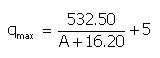﻿ Output > Empirical methods

# EMPIRICAL/UDOMETRICAL METHODS FOR HIGH FLOOD'S MAXIMUM FLOW ESTIMATION

With the empirical methods the maximum high flood's flow estimation depends on adopting a law regarding the probability distribution of peak flow’s annual maximum. Authors have searched for the best definition and approach.

# Forti’s formula

Forti proposed two formulas for the calculation of the specific flow, or the high flood unitary contribution in m³/sec Km², for surfaces below 1000 Km², and with maximum rain in 24 h of 400 mmand of 200-250 mm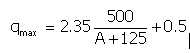# Marchi’s formula (1939)

Valid for basins with a inferior surface to 150 Km², with maximum rain in 24 h of 400 mm. The udometric coefficient will be calculated: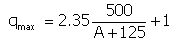# Pagliaro’s formula (1936)

Valid for basins between 20 and 1000 Km², the specific high flood maximum flow is calculated: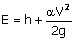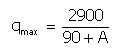where A represents basin’s surface and the flow is the specific one, or for the basin’s surface unit expressed in m³/sec Km².

# Scimeni’s formula (1928)

Valid for basin not larger than 1000 Km², the specific high flood flow is calculated: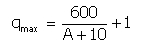where A represents basin’s surface and the flow is a specific one, or for the basin’s surface unit, expressed in m³/sec Km².

# Giandotti’s formula (1940)

Valid for basins non larger than 1000 Km², the specific flow is: